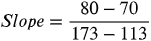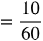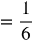1
87
views
15b
Problem

Problem 15b

Chapter 1: Functions and ModelsTextbook ExpertVerified Tutor
21 Oct 2021

Given information

As the question suggests, we have to take the temperature, T as the function of the number of chirps per minute, N.

Hence we can take N on the x-axis and T on the y-axis.

According to question,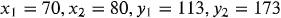Step-by-step explanation

Step 1.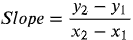Now, Plugging in the values from the question,